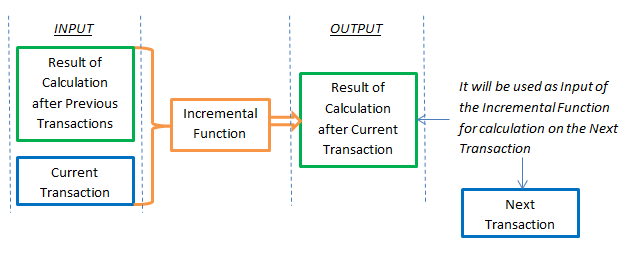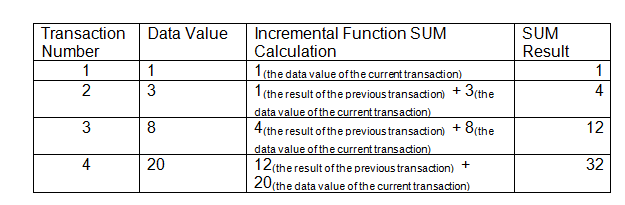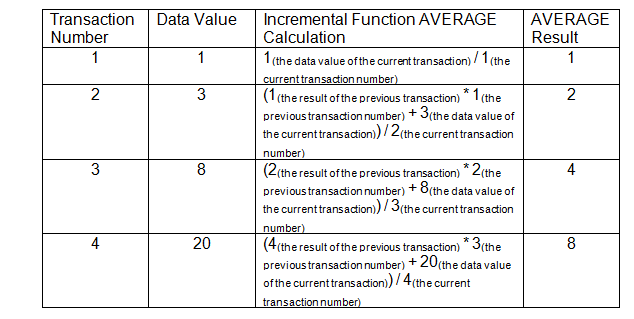# Doing better real-time calculations in the data warehouse

Share
Register now

The article “Real-time data processing is the way around the processable problem” that appeared in Information Management describes real-time data processing as the way to eliminate big processable data problem. But there is bit more to the solution than what was presented there.

The processed data should not only be stored and presented, but also used in calculations (for example, in the data warehouse). Accumulating these data will create the problem of processing large amounts of data, as described in the article mentioned above. To avoid such accumulation, the calculations must be in real time.Existing calculations in computers are usually oriented on processing accumulated data (for example, functions SUM and AVERAGE in computer languages). Real time calculations require a different type of functions.

Such functions were called incremental functions because the result of calculation is obtained incrementally, after each transaction. This differs from the calculation based on the accumulated data, when the calculation is obtained once - after all transactions.

An incremental function as an implementation of a math function, has INPUT and OUPUT. Here is how an Incremental Function calculated for the Current Transaction and creates Output for the Next transaction:The result of the incremental function, by definition, depends on the data values of the current transaction and on the result of the previous transaction, but it can also depend on the transaction numbers.

For example, calculation of the Incremental Function, which is equivalent to the regular function SUM, depends only on the data values:Another example, calculation of the Incremental Function, which is equivalent to the regular function AVERAGE, depends both on the data values and on the transaction numbers:The examples show that an incremental function for the first transaction calculated simpler than for other transactions because the first transaction does not have a previous transaction.

Incremental functions allow, for example, the opportunity to recalculate the report as soon as a new transaction is entered in the IT system and the report will always show the result in real time. Incremental functions can also be used to create real-time data warehouses and OLAP systems, where each new transaction is processed in real time, and aggregated values are recalculated in real time based on the values of the new transactions.